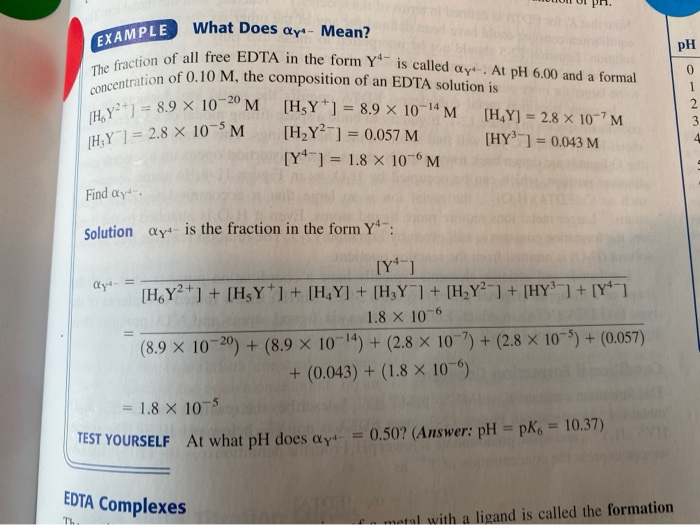# I would like to know how to solve the Test Yourself portion of the question: At...

###### Question:

I would like to know how to solve the Test Yourself portion of the question: At what pH does the fraction of EDTA in the form Y^4- = 0.50?LUNI UI PA. PH The fraction of concentration of 0.10 MPLE What Does Qy* - Mean? call free EDTA in the form Y" is called ay. At pH 6.00 and a formal tion of 0.10 M, the composition of an EDTA solution is 1 89 X 1020 M [HY'] = 8.9 X 10-4 M [HY] = 2.8 x 10-7M -1 = 2.8 X 10^M [H2Y2-1 = 0.057 M [HY) = 0.043 M [Y ] = 1.8 X 10 M Find ay. Solution ay is the fraction in the form Yº-: dy- = [Y4-1 [H.Y2+] + [H Y*1 + [H.Y] + [HRY] + [H,Y"] + [HY] + [Yº-1 1.8 X 106 (8.9 x 10-20) + (8.9 X 10-14) + (2.8 X 10-?) + (2.8 x 10-5) + (0.057) + (0.043) + (1.8 X 100) = 1.8 X 105 TEST YOURSELF At what pH does Qyt- = 0.50? (Answer: pH =pK6 = 10.37) EDTA Complexes Th matal with a ligand is called the formation

#### Similar Solved Questions

##### 2. Two quantities p and q are related as You measure p for different values of...
2. Two quantities p and q are related as You measure p for different values of q and find 3 13 4 15 5 19 6 24 7 29 8 33 (a) Identify the dependent and independent variable, and the param- eters. (b) calculate α and β, using excel...